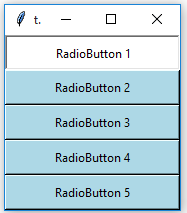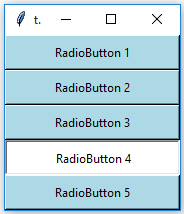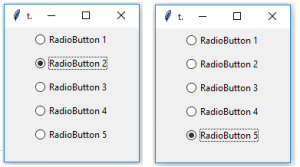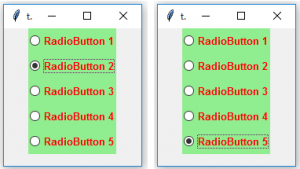GFG App
Open AppBrowser
Continue

The Radiobutton is a standard Tkinter widget used to implement one-of-many selections. Radiobuttons can contain text or images, and you can associate a Python function or method with each button. When the button is pressed, Tkinter automatically calls that function or method.
Syntax:

button = Radiobutton(master, text=”Name on Button”, variable = “shared variable”, value = “values of each button”, options = values, …)
shared variable = A Tkinter variable shared among all Radio buttons
value = each radiobutton should have different value otherwise more than 1 radiobutton will get selected.

Code #1:
Radio buttons, but not in the form of buttons, in form of button box. In order to display button box, indicatoron/indicator option should be set to 0.

## Python3

 `# Importing Tkinter module` `from` `tkinter ``import` `*` `# from tkinter.ttk import *`   `# Creating master Tkinter window` `master ``=` `Tk()` `master.geometry(``"175x175"``)`   `# Tkinter string variable` `# able to store any string value` `v ``=` `StringVar(master, ``"1"``)`   `# Dictionary to create multiple buttons` `values ``=` `{``"RadioButton 1"` `: ``"1"``,` `          ``"RadioButton 2"` `: ``"2"``,` `          ``"RadioButton 3"` `: ``"3"``,` `          ``"RadioButton 4"` `: ``"4"``,` `          ``"RadioButton 5"` `: ``"5"``}`   `# Loop is used to create multiple Radiobuttons` `# rather than creating each button separately` `for` `(text, value) ``in` `values.items():` `    ``Radiobutton(master, text ``=` `text, variable ``=` `v, ` `                ``value ``=` `value, indicator ``=` `0``,` `                ``background ``=` `"light blue"``).pack(fill ``=` `X, ipady ``=` `5``)`   `# Infinite loop can be terminated by` `# keyboard or mouse interrupt` `# or by any predefined function (destroy())` `mainloop()`

Output:The background of these button boxes is light blue. Button boxes having a white backgrounds as well as sunken are selected ones.Code #2: Changing button boxes into standard radio buttons. For this remove indicatoron option.

## Python3

 `# Importing Tkinter module ` `from` `tkinter ``import` `*` `from` `tkinter.ttk ``import` `*`   `# Creating master Tkinter window ` `master ``=` `Tk() ` `master.geometry(``"175x175"``) `   `# Tkinter string variable ` `# able to store any string value ` `v ``=` `StringVar(master, ``"1"``) `   `# Dictionary to create multiple buttons ` `values ``=` `{``"RadioButton 1"` `: ``"1"``, ` `        ``"RadioButton 2"` `: ``"2"``, ` `        ``"RadioButton 3"` `: ``"3"``, ` `        ``"RadioButton 4"` `: ``"4"``, ` `        ``"RadioButton 5"` `: ``"5"``} `   `# Loop is used to create multiple Radiobuttons ` `# rather than creating each button separately ` `for` `(text, value) ``in` `values.items(): ` `    ``Radiobutton(master, text ``=` `text, variable ``=` `v, ` `        ``value ``=` `value).pack(side ``=` `TOP, ipady ``=` `5``) `   `# Infinite loop can be terminated by ` `# keyboard or mouse interrupt ` `# or by any predefined function (destroy()) ` `mainloop() `

Output:These Radiobuttons are created using tkinter.ttk that is why background option is not available but we can use style class to do styling.

## Python3

 `# Importing Tkinter module` `from` `tkinter ``import` `*` `from` `tkinter.ttk ``import` `*`   `# Creating master Tkinter window` `master ``=` `Tk()` `master.geometry(``'175x175'``)`   `# Tkinter string variable` `# able to store any string value` `v ``=` `StringVar(master, ``"1"``)`   `# Style class to add style to Radiobutton` `# it can be used to style any ttk widget` `style ``=` `Style(master)` `style.configure(``"TRadiobutton"``, background ``=` `"light green"``, ` `                ``foreground ``=` `"red"``, font ``=` `(``"arial"``, ``10``, ``"bold"``))`   `# Dictionary to create multiple buttons` `values ``=` `{``"RadioButton 1"` `: ``"1"``,` `          ``"RadioButton 2"` `: ``"2"``,` `          ``"RadioButton 3"` `: ``"3"``,` `          ``"RadioButton 4"` `: ``"4"``,` `          ``"RadioButton 5"` `: ``"5"``}`   `# Loop is used to create multiple Radiobuttons` `# rather than creating each button separately` `for` `(text, value) ``in` `values.items():` `    ``Radiobutton(master, text ``=` `text, variable ``=` `v, ` `                ``value ``=` `value).pack(side ``=` `TOP, ipady ``=` `5``)`   `# Infinite loop can be terminated by` `# keyboard or mouse interrupt` `# or by any predefined function (destroy())` `mainloop()`

Output:You may observe that font style is changed as well as background and foreground colors are also changed. Here, TRadiobutton is used in style class, it automatically applies styling to all the available Radiobuttons.

My Personal Notes arrow_drop_up